Computational & Technology Resources
an online resource for computational,
engineering & technology publications
Civil-Comp Proceedings
ISSN 1759-3433
CCP: 84
PROCEEDINGS OF THE FIFTH INTERNATIONAL CONFERENCE ON ENGINEERING COMPUTATIONAL TECHNOLOGY
Edited by: B.H.V. Topping, G. Montero and R. Montenegro
Paper 31

A Numerical Approach for Gaussian Rational Formulas to Handle Difficult Poles

J.R. Illán González1 and G. López Lagomasino2

1Department of Applied Mathematics I, University of Vigo, Spain
2Department of Mathematics, University "Carlos III de Madrid", Spain

Full Bibliographic Reference for this paper
J.R. Illán González, G. López Lagomasino, "A Numerical Approach for Gaussian Rational Formulas to Handle Difficult Poles", in B.H.V. Topping, G. Montero, R. Montenegro, (Editors), "Proceedings of the Fifth International Conference on Engineering Computational Technology", Civil-Comp Press, Stirlingshire, UK, Paper 31, 2006. doi:10.4203/ccp.84.31
Keywords: Gauss rational quadrature formula, smoothing transformation, difficult poles, substitution mapping, meromorphic integrand.

Summary
Let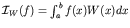. This paper shows how the technique of changing the variable to increase the efficiency of a Gaussian rational quadrature formula (GRQF) can be applied. We are especially interested in evaluating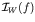when f is analytic but has poles close to [a,b]. We say that a pole of f is difficult when it is so close to [a,b] that instability shows up. We mainly consider the case in which the integrand f has real difficult poles and the weight function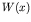possibly has integrable singularities at the end points of the integration interval.

Gautschi  has developed routines to calculate nodes and coefficients for a GRQF when some poles of f are difficult. The authors and Fidalgo  found a method different from Gautschi's which has been successfully applied to compute simultaneous rational quadrature formulas (SRQF). This paper presents a version of the SRQF approach adapted to GRQF for evaluating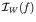efficiently. Basically, the procedure consists in the use of a GRQF which is exact for rational functions whose poles are those of the integrand which are difficult.

Let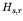be the array defined as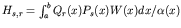,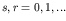, where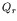, is the r-th orthogonal polynomial associated to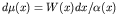,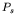is a suitable polynomial with zeros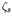and degree s, and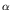is a polynomial whose zeros simulate difficult poles of the integrand f.

The rational function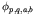given below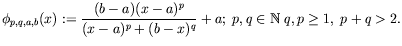(62)

is adopted as the smoothing transformation of [a,b] which is fitted into the modified moments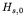. One expects that the process of changing the variable produces the effect of moving real poles away from I. The singularities of W are also annihilated, so that the integralscan be computed accurately by applying a composite Gauss-Legendre rule.

If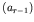and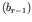are the coefficients of the recurrence relation which the polynomialsfulfil, then we can easily derive a relationship as follows: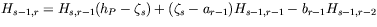(63)

Thus, we only have to calculate the moments,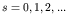, to obtain, step by step, all the recurrence coefficients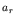,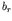,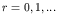For every r the corresponding Jacobi's matrix provides the nodes and coefficients of the GRQF of order r.

Table 1 is a sample of the results produced when the following integral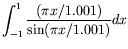(64)

is evaluated by the smoothing method and by Gautschi's. In comparison, one observes that this approach is superior to that reported in .

 Order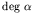A B OrderA B 3 2 2.2e-03 4.1e-01 2 1 3.9e-01 4.1e-01 8 2 4.5e-09 9.3e-06 4 2 1.7e-04 2.9e-02 6 4 1.3e-08 8.4e-03 6 3 8.2e-07 2.1e-03 9 6 9.6e-16 1.1e-04 8 4 1.2e-11 1.2e-05 12 8 8.2e-15 6.0e-07 10 5 6.9e-16 7.3e-06

We present a slight variant of the smoothing method to improve the accuracy when some poles are very difficult, that is, when the distance from the pole to the integration interval is less than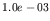. It consists of fitting a substitution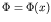,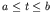, into. This mapping is defined as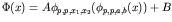, where p is even,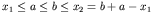,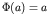and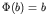.

One of the experimental conclusions is that the smoothing method also works when the non real difficult poles are in the region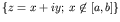.

The results of some numerical tests are shown in the paper to illustrate the power of this approach when they are compared with those produced by other polynomial and rational methods.

References
1
W. Gautschi, "Algorithm 793: GQRAT-Gauss quadrature for rational functions", ACM Trans. Math. Software, 25, 213-239, 1999. doi:10.1145/317275.317282
2
U. Fidalgo Prieto, J.R. Illán González and G. López Lagomasino, "Convergence and computation of simultaneous rational quadrature formulas", Submitted to Numer. Math. doi:10.1007/s00211-006-0056-8

purchase the full-text of this paper (price £20)